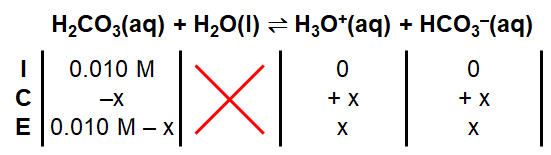# Problem: Calculate [CO32-] in a 0.010-M solution of CO2 in water (usually written as H2CO3). If all the CO32- in this solution comes from the reactionHCO3-(aq) ⇌ H+(aq) + CO32-(aq)what percentage of the H+ ions in the solution is a result of the dissociation of HCO3-? When acid is added to a solution of sodium hydrogen carbonate (NaHCO3), vigorous bubbling occurs. How is this reaction related to the existence of carbonic acid (H2CO3) molecules in aqueous solution?

🤓 Based on our data, we think this question is relevant for Professor Liwosz's class at UB.

###### FREE Expert Solution

Given: 0.010 M HCO3               Ka1 = 4.5 × 10–7               Ka2 = 4.7 × 10–11

Part A:

ICE Chart for Ka1:###### Problem Details

Calculate [CO32-] in a 0.010-M solution of CO2 in water (usually written as H2CO3). If all the CO32- in this solution comes from the reaction

HCO3-(aq) ⇌ H+(aq) + CO32-(aq)

what percentage of the H+ ions in the solution is a result of the dissociation of HCO3-? When acid is added to a solution of sodium hydrogen carbonate (NaHCO3), vigorous bubbling occurs. How is this reaction related to the existence of carbonic acid (H2CO3) molecules in aqueous solution?